Division Of Fraction By Whole Number  by  Prisca Mwikya

What is the reciprocal of the following numbers.

2,3,4,5,6,7,8,9,10?

• Reciprocal is what you multiply a value by to get 1.

• Its called multiplicative inverted.

• This simply means, turning number up- side -down.

• Remember whole numbers are always the numerator while 1is the denominator.

• Therefore 2/1=1/2, 3/1=1/3,4/1=1/4,5/1 =1/5

• 6/1 =1/6, 7/1=1/7 ,8/1 =1/8, 9/1= 1/9 and , 10/1 =1/10

Example 1.

Work out divide 1/ 3 by 8

Get the reciprocal of 8 which Will be 1/8 since 8 = 8/1

Replace the symbol of division with multiplication symbol

Therefore 1/3/8 will be ; 1/3x1/8 = 1/24

Example 2

Alice divided a half an orange between her three children.What part of an orange did each child get?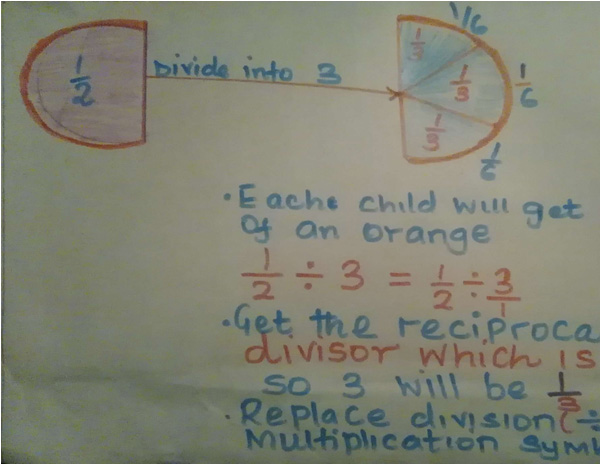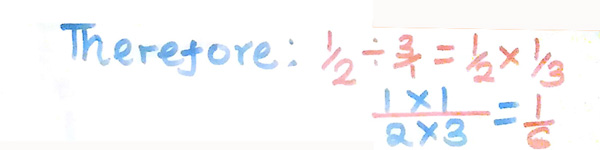Example 3

Work out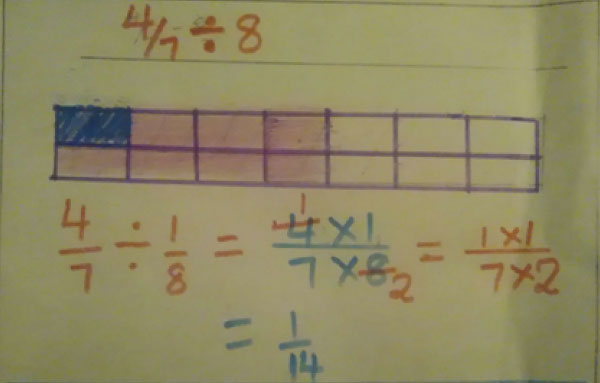Example 4.

Work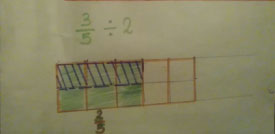Example 4.

Work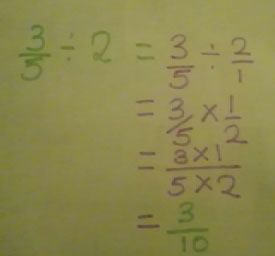Exercise 1.

Work out the following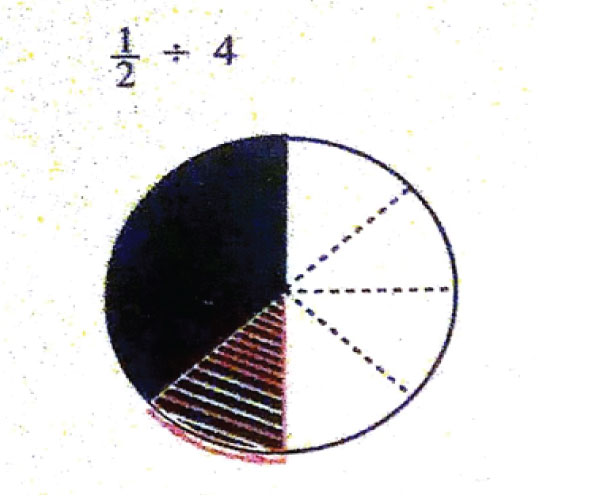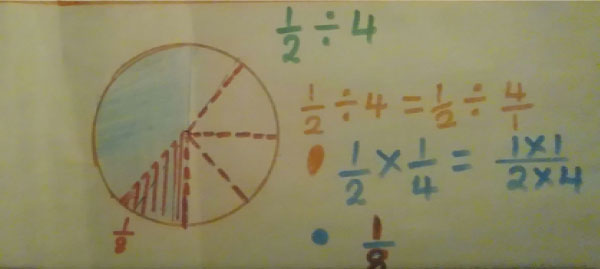2.Work out

3.Work out

Nyabera bought  3/4 m of a cloth.She divided it into three equal parts and made baby pants from each piece.What fraction of a metre did each part take?

Division of a whole a number by a fraction

(i) instead of dividing thenumber multiply it inverse (reciprocal) .
(ii) change the operation to multiplication and the divisor to reciprocal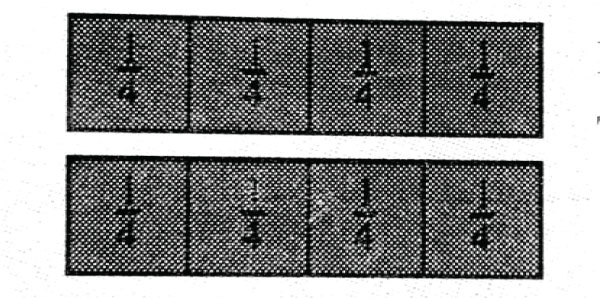6

Example 2How many thirds are in two oranges ?

Exercise 2

• Work out the following

4. A sack of sugar contains 90 kg.The sugar is repackaged into 1\4 kg packets.How many packets were obtained?

1. 10
2. 10
3. 5
4. 360
5. 4

6

LESSON 36 MATHEMATICS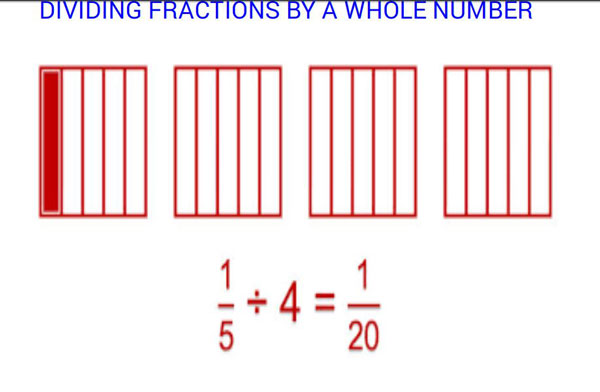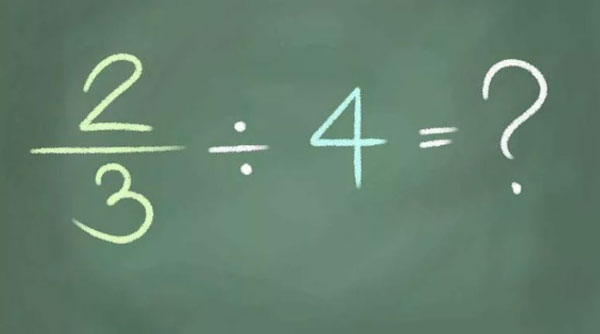Write the whole number as Fraction.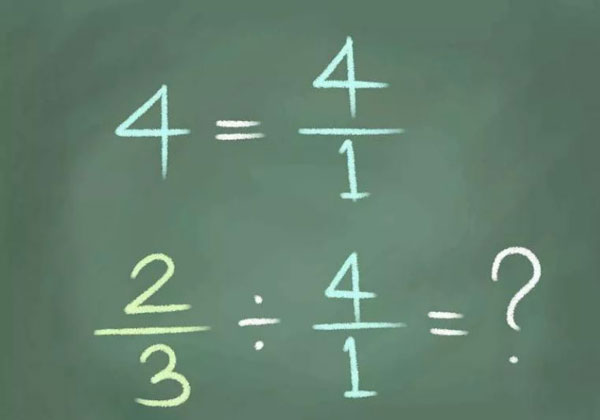Change division to multiplication.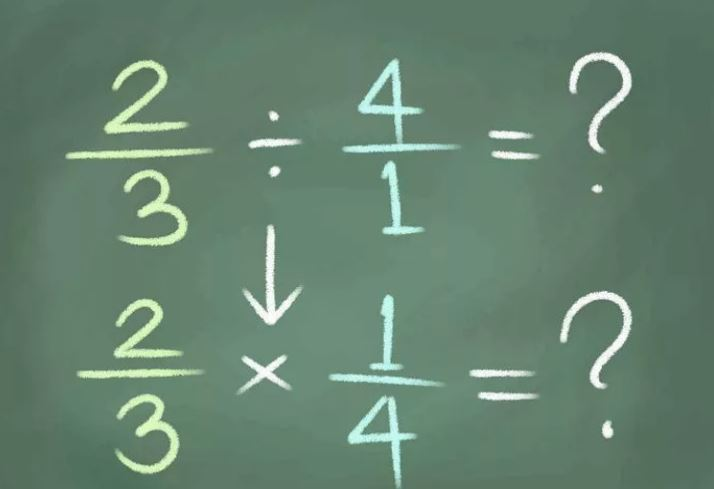Multiply by the reciprocal of the whole number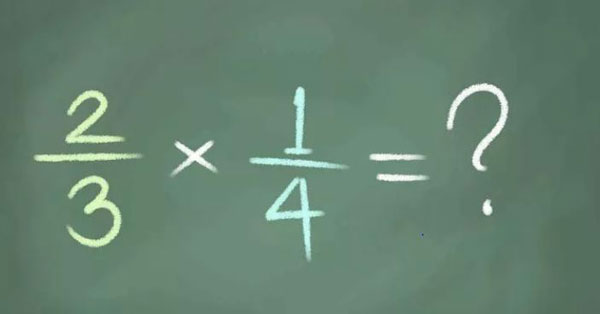Multiply the numerators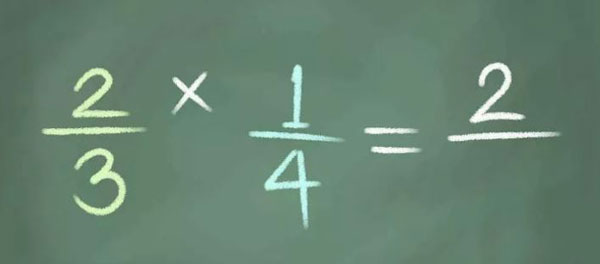Multiply the denominators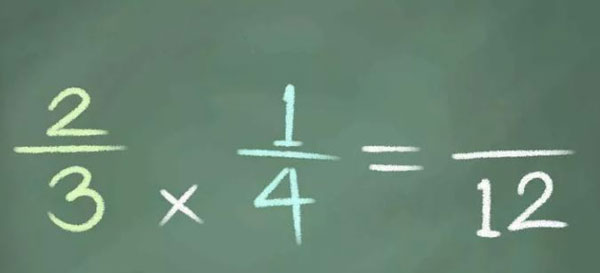Simplify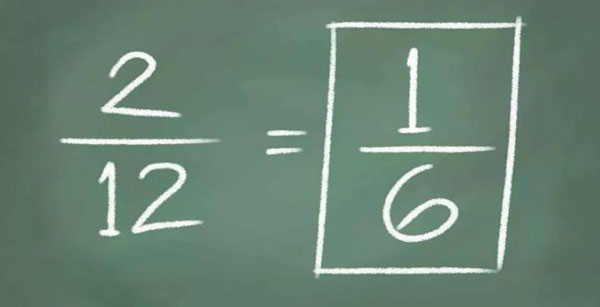EXERCISE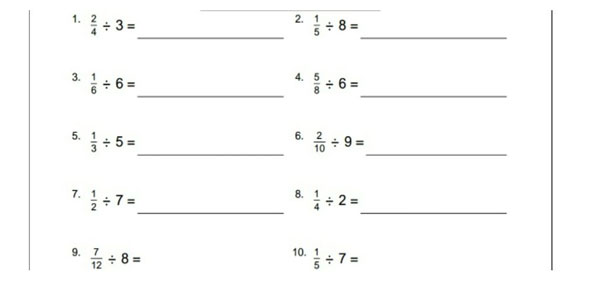### Sources

• DF_I-1 by elimu used under CC_BY-SA
• DF_img2 by elimu used under CC_BY-SA
• DF_IMG3 by elimu used under CC_BY-SA
• DF_IMG4 by elimu used under CC_BY-SA
• DF_IMG5 by elimu used under CC_BY-SA
• DF_IMG6 by elimu used under CC_BY-SA
• DF_IMG7 by elimu used under CC_BY-SA
• DF_IMG8 by elimu used under CC_BY-SA
• DF_IMG9 by elimu used under CC_BY-SA
• 0c0932b0-7071-4f57-8f36-3262fc5efdf5 by google photo used under CC_BY
• 38c153a9-f8c9-4db0-b1ce-f619419e1a43 by google photo used under CC_BY
• 3af3dafe-df7f-4f1c-8a2c-0b4141802f1e by google photo used under CC_BY
•